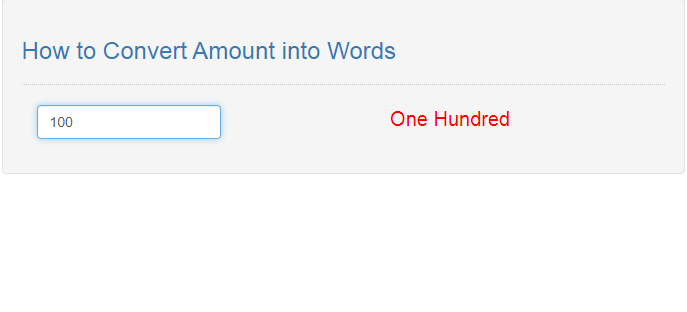# How to Convert Amount into Words in JavaScript

## Introduction

In this tutorial we will create a How to Convert Amount into Words in JavaScript. This tutorial purpose is to make an automatic feature that will convert the enter amount into word. This will cover all the important function that will make this program works. I will provide a sample program to show the actual coding of this tutorial.

This tutorial is simple and easy to understand just follow the instruction I provided and you can do it without a problem. This program is useful to any system that needed to enter a numbers or generating receipt. I will give my best to provide you the easiest way of creating this program Converting Amount into Words. So let's do the coding.

## Before we get started:

This is the link for the template that i used for the layout design https://getbootstrap.com/.

## Creating The Interface

This is where we will create a simple interface for our application. This code will display only a textbox that will be need to enter your integer value. To create this simply copy and write it into your text editor, then save it as index.html.
`<!DOCTYPE html><html lang="en">	<head>		<meta charset="UTF-8" name="viewport" content="width=device-width, initial-scale=1"/>		<link rel="stylesheet" type="text/css" href="css/bootstrap.css"/>	</head><body>	<nav class="navbar navbar-default">		<div class="container-fluid">			<a class="navbar-brand" href="https://sourcecodester.com">Sourcecodester</a>		</div>	</nav>	<div class="col-md-3"></div>	<div class="col-md-6 well">		<h3 class="text-primary">How to Convert Amount into Words</h3>		<hr style="border-top:1px dotted #ccc;">		<div class="col-md-4">			<div class="form-group">				<input type="text" class="form-control" maxlength="6" onkeypress="return NumberInput(this.value);" placeholder="Enter amount" onkeyup="NumberToWords(this.value);"/>			</div>		</div>		<div class="col-md-8"> 			<center><div id="result" style="color:#ff0000; font-size:20px;"></div></center>		</div>	</div></body><script src="script.js"></script></html>`

## Creating JavaScript Function

This is where the main function of the application is. This code will automatically convert the entered amount into words dynamically. To do this just copy and write these block of codes inside the text editor and save it as script.js.
`function NumberToWords(number){	var num = new String(number);	var splt = num.split("");	var newNumber = splt.reverse();	var first = ['Zero', 'One', 'Two', 'Three', 'Four', 'Five', 'Six', 'Seven', 'Eight', 'Nine'];	var two = ['Ten', 'Eleven', 'Twelve', 'Thirteen', 'Fourteen', 'Fifteen', 'Sixteen', 'Seventeen', 'Eighteen', 'Nineteen'];	var tens = ['', 'Ten', 'Twenty ', 'Thirty ', 'Forty ', 'Fifty ', 'Sixty ', 'Seventy ', 'Eighty ', 'Ninety ']; 	var word = new Array();	var count = 0; 	for(i=0; i < newNumber.length; i++){		switch(i){			case 0:               if ((newNumber[i] == 0) || (newNumber[i + 1] == 1)) {                   word[count] = '';               }               else {                   word[count] = first[newNumber[i]];               } 			word[count] = word[count];                break; 			case 1:				Tens();               break; 			case 2:				if (newNumber[i] == 0) {					word[count] = '';				}				else if ((newNumber[i - 1] == 0) || (newNumber[i - 2] == 0)) {					word[count] = first[newNumber[i]] + " Hundred ";				}				else {					word[count] = first[newNumber[i]] + " Hundred ";				}               break;  			case 3:				if (newNumber[i] == 0 || newNumber[i + 1] == 1) {					word[count] = '';				}				else {					word[count] = first[newNumber[i]];				}				if ((newNumber[i + 1] != 0) || (newNumber[i] > 0)) {					word[count] = word[count] + " Thousand ";				}               break; 			case 4:				Tens();               break; 			case 5:				if ((newNumber[i] == 0) || (newNumber[i + 1] == 1)) {                   word[count] = '';				}				else {					word[count] = first[newNumber[i]];				}				if (newNumber[i + 1] !== '0' || newNumber[i] > '0') { 					if(newNumber === "0" && newNumber === '0'){						word[count] = word[count] + " Hundred Thousand ";						}else{						word[count] = word[count] + " Hundred ";					}				}                break;  			default: break;		} 		count++; 	}  	function Tens() {		if (newNumber[i] == 0) { 			word[count] = ''; 		}else if (newNumber[i] == 1) { 			word[count] = two[newNumber[i - 1]]; 		}else { 			word[count] = tens[newNumber[i]]; 		}	} 	word.reverse();	var result = '';	for(i=0; i < newNumber.length; i++){		result = result + word[i];	}  	document.getElementById('result').innerHTML = result;  } function NumberInput(evt) {	var e = event || evt;	var charCode = e.which || e.keyCode; 	if (charCode > 31 && (charCode < 48 || charCode > 57))		return false;	return true;}`

In the code above we first create a method called NumberToWords() and set several variables in the first couple of lines. Then we use switch statement in order to make it easier for use to set several function into it. We use a lot of if statement to determine the value of total length of the entered amount.

We then create a special function that will track the entered value if it is a tenths value or not called Tens(). Lastly we create a special method that will only track the keyboard if the value that are entered is only number, we called it NumberInput()

## Output: